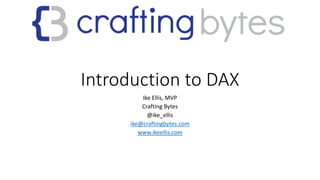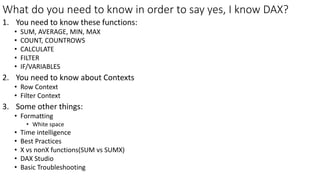Successfully reported this slideshow.

# Introduction to DAX

7

Share×
1 of 39
1 of 39

# Introduction to DAX

7

Share

Power BI, SSAS Tabular, and Excel all use DAX. This presentation is meant to be used with a PBIX notebook found here: https://github.com/IkeEllis/democode/blob/master/IntroToDAX/Power%20BI%20Introduction%20to%20DAX.pbix

Power BI, SSAS Tabular, and Excel all use DAX. This presentation is meant to be used with a PBIX notebook found here: https://github.com/IkeEllis/democode/blob/master/IntroToDAX/Power%20BI%20Introduction%20to%20DAX.pbix

## More Related Content

### Related Books

Free with a 14 day trial from Scribd

See all

### Related Audiobooks

Free with a 14 day trial from Scribd

See all

### Introduction to DAX

1. 1. Introduction to DAX Ike Ellis, MVP Crafting Bytes @ike_ellis ike@craftingbytes.com www.ikeellis.com
2. 2. Do you know DAX?
3. 3. What do you need to know in order to say yes, I know DAX? 1. You need to know these functions: • SUM, AVERAGE, MIN, MAX • COUNT, COUNTROWS • CALCULATE • FILTER • IF/VARIABLES 2. You need to know about Contexts • Row Context • Filter Context 3. Some other things: • Formatting • White space • Time intelligence • Best Practices • X vs nonX functions(SUM vs SUMX) • DAX Studio • Basic Troubleshooting
4. 4. So how will you learn it? • Go in order: • This slide deck • This Power BI Project • Practice, practice, practice
5. 5. And how will you use it? • Works in Excel • Works in Power BI • Works in SQL Server Analysis Services (SSAS) Tabular
6. 6. This presentation • Simplifies the complexity • Might not tell you the whole truth for the sake of simplicity • Is not seeking to be comprehensive, but instead, seeks to allow you to answer the question, yes, I know DAX in that job interview
7. 7. Your first DAX expression • Check Power BI Desktop tab “Your First DAX Expression” • It’s a calculated column called Order Line Total • Meant to add a column to every record in a table. Order Line Total = 'Sales OrderDetails'[qty] * 'Sales OrderDetails'[unitprice]
8. 8. Your Second DAX Expression • Check Power BI Desktop tab “Your Second DAX Expression” • This is a measure • Meant to perform an aggregation that we can slice & dice Total Sales = SUM('Sales OrderDetails'[Order Line Total])
9. 9. How can we verify this? select sum(Sales.OrderDetails.unitprice * Sales.OrderDetails.qty) from sales.OrderDetails
10. 10. DAX Expression Breakdown Name the measure. You’ll use that in the visualization Built in DAX formula Table name Column nameEquals sign separates expression name from expression formula Total Sales = SUM('Sales OrderDetails'[Order Line Total])
11. 11. All kinds of easy to use DAX formulas that you can learn quickly • SUM • AVERAGE • MIN • MAX • COUNT • COUNTROWS • DATEDIFF • DATEADD
12. 12. • Look at tab “Easy DAX built-in formulas” • Look under the Orders table for the calculated column “Days to Ship” • Look under Orders table for measure “Average Days to Ship Days To Ship = DATEDIFF('Sales Orders'[orderdate], 'Sales Orders'[shippeddate],DAY) Play again: AVERAGE and DATEDIFF Average Days to Ship = AVERAGE('Sales Orders'[Days To Ship])
13. 13. Your Third DAX expression: Calculated Table • Look at the Dates table. It was built with a DAX expression • Created a Dates table with a date per day between the specified range • Also created a Dates hierarchy Dates = CALENDAR("1/1/2000", "12/31/2016")
14. 14. Now on to Contexts! • Two different contexts: • Row Context • Filter Context
15. 15. Row Context • We already know how this works! We’ve been using it for all of our calculated columns. Let’s revisit our first DAX Expression • Notice we expect a value per row in a table • This runs at import and gets stored • Might increase file size Order Line Total = 'Sales OrderDetails'[qty] * 'Sales OrderDetails'[unitprice]
16. 16. Filter Context • Easy to show with measures • Look at Filter Context 1, 2, 3 in the Power BI Desktop file (PBIX).
17. 17. Filter Context 1 • We see it filtered by year and optionally by product category • The measure is only defined once, and the DAX engine takes care of doing the calculations on the fly • The calculations are not stored, but created and retrieved at query time
18. 18. Filter Context 2 Total Sales by year and with a page filter.
19. 19. Filter Context 3 • Visuals can impact each other and change context
20. 20. Now that we understand context, we can answer this: Measures vs Calculated Columns Measures Calculated Columns Different functions Uses Row Contex Doesn’t take up space Mostly text Less space Executes at the point data is read into the model and saved (value is static) Filter Context Executed at the time it is used
21. 21. CALCULATE: Breaking out of the filter context Beverages Total Sales = CALCULATE ( SUM('Sales OrderDetails'[Order Line Total]) , 'Production Categories'[categoryname] = "Beverages" ) AGGREGATION FILTER Look at the tab CALCULATE
22. 22. FILTER Number of Orders = COUNT('Sales Orders'[orderid]) Number of US Orders = CALCULATE ( COUNT ( 'Sales OrderDetails'[orderid] ) , FILTER ( 'Sales Customers' , 'Sales Customers'[country] = "USA" ) )
23. 23. VARIABLES & RETURN Total Sales For Customers with Minimum Order Count = VAR MinimumOrderCount = 5 VAR CustomersWithMinimumOrders = CALCULATE ( sum('Sales OrderDetails'[Order Line Total]) , FILTER('Sales Customers', [Number of Orders] > MinimumOrderCount) ) RETURN CustomersWithMinimumOrders
24. 24. Variables VAR myVar = 1 Data Type Variable Name Variable Value RETURN myVar + 25 Expressions must use RETURN to return a value
25. 25. Debugging using variables • RETURN does not need to return the last variable • In a multi-step formula, you can return an earlier value to troubleshoot it • Simplifies the reading of code, rather than endlessly nesting values over and over again.
26. 26. Time Intelligence: TOTALYTD YTD Total Sales = TOTALYTD ( SUM('Sales OrderDetails'[Order Line Total]) , Dates[Date].[Date] )
27. 27. Time Intelligence: PREVIOUSMONTH Total Sales Previous Month = CALCULATE ( sum('Sales OrderDetails'[Order Line Total]) , PREVIOUSMONTH(Dates[Date]) )
28. 28. X vs nonX functions(SUM vs SUMX) • SUM is an aggregator function. It works like a measure, calculating based on the current filter context. • SUMX is an iterator function. It works row by row. SUMX has awareness of rows in a table, hince can reference the intersection of each row with any columns in the table.
29. 29. SUM vs SUMX Example Total Sales SUMX = SUMX( 'Sales OrderDetails' , 'Sales OrderDetails'[qty] * 'Sales OrderDetails'[unitprice] ) Total Sales = SUM('Sales OrderDetails'[Order Line Total])
30. 30. Best Practice: Organize your code • Keep measures together • Organize them by type • Simple aggregation • Time variance • Ratios and differences • Business-specific calculations
31. 31. Best Practice: Naming Columns & Measures • Feel free to use spaces • Avoid acronyms • Make names terse, but descriptive • Makes Q & A easier to use • In formulas, reference table names for calculated columns and do not reference table names for measures, so you’ll know the difference
32. 32. Best Practice: Formatting • DAX Expressions can have lots of parentheses and square brackets • Please use white space to control this • Here’s an example of a properly formatted calculated column Days To Ship = DATEDIFF ( 'Sales Orders'[orderdate] , 'Sales Orders'[shippeddate] , DAY )
33. 33. Basic Troubleshooting • A lot of things can go wrong, but the problem is usually one of two things: 1. A relationship is misconfigured or the data is wrong in the model. 2. Wrong data type
34. 34. Data types • Numeric • String • Bool • DateTime • If a function is expecting a numeric, but gets a string, it won’t work. Clean up the model and watch it start working. • Uses less space and memory with your model • Improves performance
35. 35. Relationships
36. 36. Manipulating the relationships Total Sales By Ship Year = CALCULATE ( SUM('Sales OrderDetails'[Order Line Total]) , USERELATIONSHIP('Sales Orders'[shippeddate], Dates[Date]) ) Only one active relationship at a time
37. 37. DAX Studio • Parses • Formats • Shows execution plan • Connects to SSAS Tabular or Power BI Desktop
38. 38. Other Resources
39. 39. Contact Me! http://www.craftingbytes.com http://blog.ikeellis.com http://www.ikeellis.com YouTube http://www.youtube.com/user/IkeEllisData San Diego Tech Immersion Group http://www.sdtig.com Twitter: @ike_ellis 619.922.9801 ike@craftingbytes.com